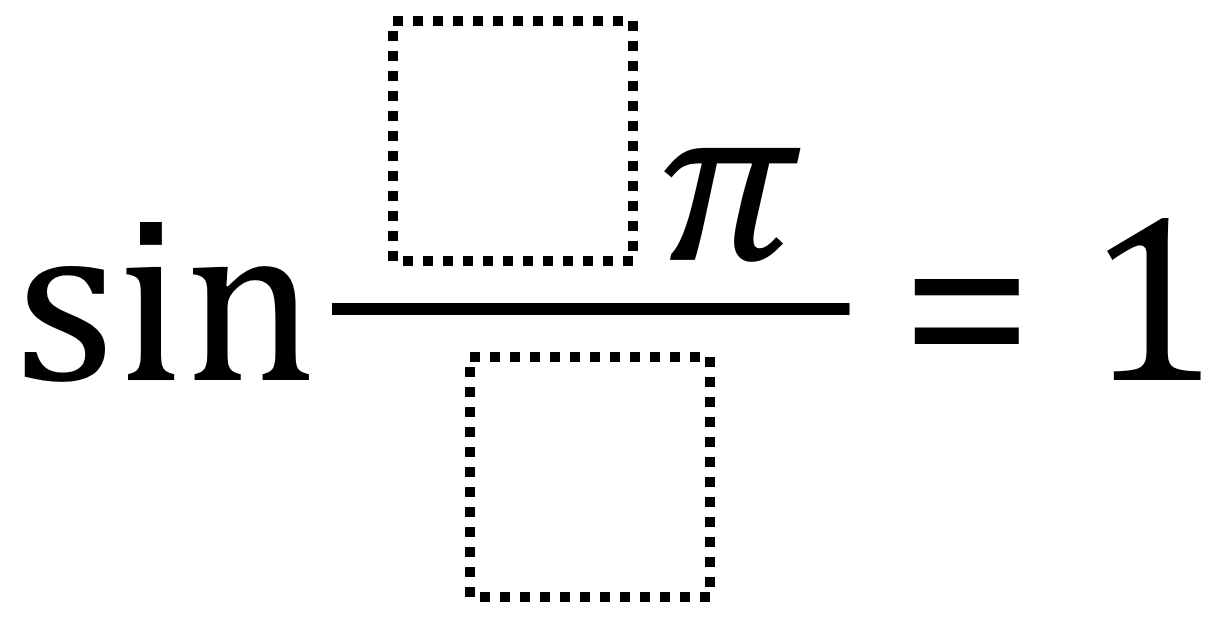Home > High School: Functions > Sine Functions

# Sine Functions

Directions: Use the digits 1 to 9, at most one time each, to fill in the boxes and make two true number sentences.### Hint

When is the sine of a value equal to 1?
How does sine being a periodic function affect the potential values?

### Answer

The obvious answer is 1*pi/2. The problem’s intent is for students to realize that having a period of 2pi means that sine(pi/2 + 2pi + 2pi + …) will also equal 1. So, answers also include 5*pi/2 and 9*pi/2.

Some other potential answers, that are also worth discussing, are values that are equivalent to 1*pi/2 including 2*pi/4, 3*pi/6, and 4*pi/8.

Source: Robert Kaplinsky

## Creating Sequences

Directions: Using the digits 0-9, at most one time each, complete the first three terms …